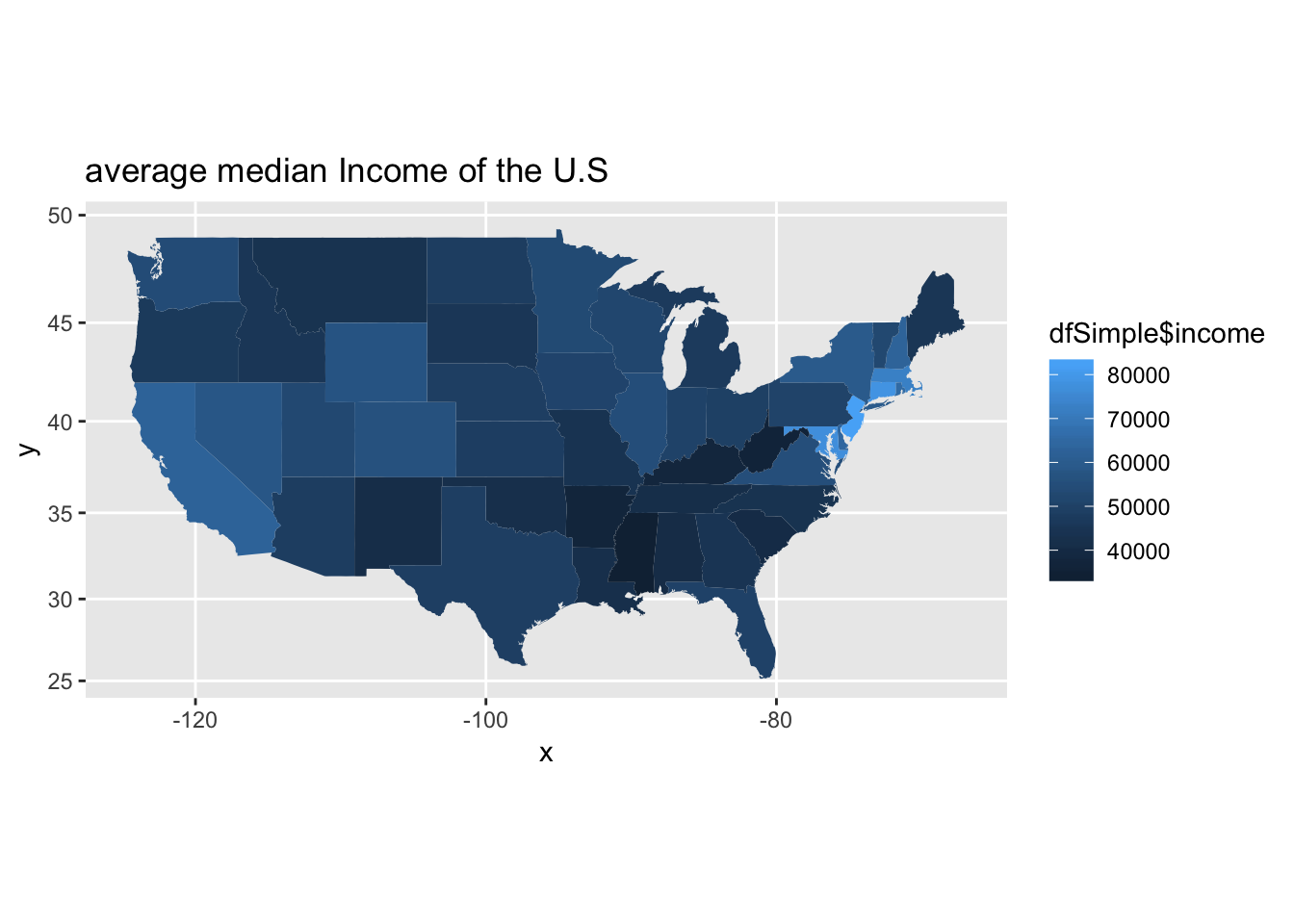# Step 1: Load the Data

``````library(gdata)
# read in the excel file using read.xls function in gdata package
mydata <- read_excel("~/Dropbox/Applied Data Science (IST 687)/Datasets/MedianZIP.xlsx")
# 2) clean up the data
# change column names to "zip", "Median","Mean", and "Population"
colnames(mydata) <- c("zip", "Median", "Mean", "Population")

# delete the first row of the dataframe
mydata <- mydata[-1,]

# gsub function is used to perform replacement of matches determined by regular expression matching
numberize <- function(v)
{
v <- gsub(",", "", v)
v <- as.numeric(v)
}

mydata <- data.frame(sapply(mydata, numberize))

# 3)  Load the "zipcodez" package
# install.packages("zipcode")
library(zipcode)
# load a dataframe that contains city, state, latitude, and longitude for U.S. ZIP codes
data(zipcode)
# clean up and standardize ZIP codes in mydata
mydata\$zip <- clean.zipcodes(mydata\$zip)

# 4)  Merge the zip code infoxrmation
# merge mydata and zipcode by the common column "zip" and store the new dataframe into "dfNew"
dfNew <- merge(mydata, zipcode, by="zip")

# 5)  Remove Hawaii and Alaska
# remove Hawaii and Alaska from dfNew
dfNew <- dfNew[which(dfNew\$state != "AK" & dfNew\$state != "HI"), ]``````

# Step 2: Show the income & population per state

`````` # 1) Create a simpler dataframe with just the average median income and the population for each state

# use tapply  to calculate average median income for each state
# and store the results in a new dataframe called "medianIncome"
income <- tapply(dfNew\$Median, dfNew\$state, mean )
state <- rownames(income)
medianIncome <- data.frame(state, income)

#calculate total popuation in each state
pop <- tapply(dfNew\$Population, dfNew\$state, sum )
state <- rownames(pop)
statePop <- data.frame(state, pop)

# merge "medianIncome" and "statePop" by common column "states"
dfSimple <- merge(medianIncome, statePop, by="state")

# 2) Add the state abbreviations and the state names as new columns
#get the state name (not just the abbreviations)
dfSimple\$stateName <- state.name[match(dfSimple\$state,state.abb)]
# convert "stateName" to lower case
dfSimple\$stateName <- tolower(dfSimple\$stateName)

# 3) Show the U.S. map, representing the color with the average median income of that state
# install.packages("ggplot2")
library(ggplot2)``````
``## Warning: package 'ggplot2' was built under R version 3.5.2``
``````  # install.packages("ggmap")
library(ggmap)``````
``## Google's Terms of Service: https://cloud.google.com/maps-platform/terms/.``
``## Please cite ggmap if you use it! See citation("ggmap") for details.``
``````  # get the data on the "state" to be mapped
us <- map_data("state")

# use "dfSimple" to create map and set "stateName" as map ID
mapIncome <- ggplot(dfSimple, aes(map_id = stateName))
# represent the color with average median income of each states
mapIncome <- mapIncome + geom_map(map = us, aes(fill = dfSimple\$income))
# change the limits of x and y axes to print the whole map
mapIncome <- mapIncome + expand_limits(x = us\$long, y = us\$lat)
# make sure the map is not stretched
mapIncome <- mapIncome + coord_map()
# add a tile for the map
mapIncome <- mapIncome + ggtitle("average median Income of the U.S")
# plot the income map
mapIncome````````````  # 4) Show the U.S. map, with color representing the population of the state
# use "dfSimple" to create map and set "stateName" as map ID
mapPop <- ggplot(dfSimple, aes(map_id = stateName))
# represent the color with population of each states
mapPop <- mapPop + geom_map(map=us, aes(fill = dfSimple\$pop))
# change the limits of x and y axes to print the whole map
mapPop <- mapPop + expand_limits(x = us\$long, y = us\$lat)
# make sure the map is not stretched and add a title for the map
mapPop <- mapPop + coord_map() + ggtitle("Population of the U.S")
# plot the map
mapPop``````